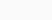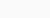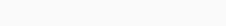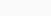Breaking News

# The highest oxidation number of Mn is found in compounds

Welcome to Catalytic, a blog to discuss the discussion of subject matter from Higher Education, High School, Middle School and Elementary Schools. This time Katalistiwa will discuss a question that is often asked in school exams. The questions are: The highest oxidation number of Mn is found in compounds

Daftar Isi## The highest oxidation number of Mn is found in compounds

a. MnCl2
b. K2MnO4
c. Mn2(SO4)3
e.Mn(NO3)2

The highest Mn oxidation number of +6 is found in potassium manganate (K₂MnO₄).

The highest oxidation number of Mn is found in the compound
a. MnCl₂ ⇒ manganese chloride
b. K₂MnO₄ ⇒ potassium manganate
c. Mn₂(SO₄)₃ ⇒ manganese (III) sulfate
d. Mn(NO₃)₂ ⇒ manganese(II) nitrate

### Detailed discussion of the Mn Oxidation Number

Before we look up the oxidation number of Mn in each of the above compounds, it is necessary to recall some of the oxidation numbers of the elements involved, as follows.
1. The oxidation number of group IA elements (alkali metals) is +1.
Thus the oxidation number of K is +1.
2. The oxidation number of group VIIA elements (halogens, non-metals) that are not bound to O (oxygen) is -1.
So the oxidation number of Cl is -1.
3. Compound ions, namely sulfate ionsIt has a total oxidation number according to its charge, which is -2.
4. Ionic compounds, namely nitrate ionsIt has a total oxidation number according to its charge, which is -1.
5. The total oxidation number of neutral compounds is zero.

#### Work on finding the oxidation number (bilox) of Mn

a. MnCl₂
Mn oxidation state + 2(Cl oxidation state) = 0
Bioxal Mn + 2(-1) = 0
∴b. K₂MnO₄
2(K oxidation state) + Mn oxidation state + 4(O oxidation state) = 0
2(+1) + oxidation state of Mn + 4(-2) = 0
2 +bioxal Mn – 8 =0
Bilox of Mn = 8 – 2
∴c. Mn₂(SO₄)₃
2(the oxidation state of Mn)+3(the oxidation state of SO₄) =0
2(biloxMn)+ 3(-2) = 0
2(bioxal Mn)=6
∴d. Mn(NO₃)₂
The oxidation state of Mn +2(the oxidation state of NO₃) =0
Bioxal Mn+2(-1) =0
BiloxMn -2 =0
∴Pay attention to questions c and d, it can also be seen as follows. For a neutral compound with the formula chemicalindex n is the charge or oxidation number of A, while index m is the charge or oxidation number of B.
Let's repeat.
c. Mn₂(SO₄)₃
Immediately seen that the oxidation number of sulfate ion compounds is -2 and the oxidation number of Mn is +3.

d. Mn(NO₃)₂
It is immediately seen that the oxidation number of the nitrate ion compound is -1 while the oxidation number of Mn is +2.